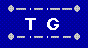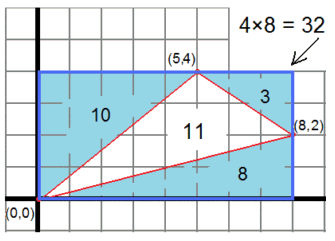Triangle Area Calculator Home  | Send Your Feedback

 Enter the (x, y) coordinates of three points, and the area of the triangle they enclose will be displayed. (x1, y1): (x2, y2):     (x3, y3): If the points define a horizontal or vertical line, the area is easily computed from 1/2 * base * height. Otherwise, an enclosing rectangle is imagined, and three right triangles outside the given triangle (and in some cases a rectangle as well) are subtracted from that to get the area of the triangle. This is much simpler than using trigonometric functions or Heron's formula. Here is an example: The points are (0,0), (5,4), and (8,2). The three right triangles have areas of (5*4)/2 = 10, (2*3)/2 = 3, and (8*2)/2 = 8. Those total 21, which when subtracted from the enclosing 4 * 8 = 32 rectangle, leaves an area of 11 for the given triangle.